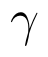Select Page

# CBSE Maths 12 Science MCQ Application of Derivatives Solutions in English

CBSE Maths 12 Science MCQ Application of Derivatives Solutions in English to enable students to get Solutions in a narrative video format for the specific question.

Expert Teacher provides CBSE Maths 12 Science MCQ Application of Derivatives Solutions through Video Solutions in English language. This video solution will be useful for students to understand how to write an answer in exam in order to score more marks. This teacher uses a narrative style for a question from Application of Derivatives not only to explain the proper method of answering question, but deriving right answer too.

Please find the question below and view the Solution in a narrative video format.

Question:

Solution Video in English:

You can select video Solutions from other languages also. Please check Solutions in ( Hindi )

## Similar Questions from CBSE, 12th Science, Maths, Application of Derivatives

Question 1 : Find the approximate change in the surface area of a cube of side x meters caused by decreasing the side by 1%. (View Answer Video)

Question 2 : The maximum value ofis, (View Answer Video)

Question 3 : The normal at the point (1, 1) on the curveis: (View Answer Video)

Question 4 : The slope of the normal to the curveat x = 0 is: (View Answer Video)

Question 5 : It is given that  at x=1, the function attainsits maximum value on the interval[0,2]. Find the value of a? (View Answer Video)

### Three Dimensional Geometry

Question 1 : If a line makes angleandwith the coordinate axis, then find the value of(View Answer Video)

Question 2 : The cartesian equation of a line is, write its vector form. (View Answer Video)

Question 3 : Find the distance between the point (7, 2, 4) and the plane determined by the points A(2, 5, -3), B(-2, -3, 5) and C(5, 3, -3). (View Answer Video)

Question 4 : Find the distance of the point (-1, -5, -10) from the point of intersection of the line joining the points A(2, -1, 2) and B(5, 3, 4) with the plane x - y + z = 5. (View Answer Video)

Question 5 : Find the vector equation of a plane which is at a distance of 5 units from the origin and its normal vector is(View Answer Video)

### Matrices

Question 1 : If,find the value of x. (View Answer Video)

Question 2 : Find the value of c from the equation:(View Answer Video)

Question 3 : Find the transpose of the matrix:. (View Answer Video)

Question 4 : If,find the value of y.  (View Answer Video)

Question 5 : Given,, find the value of x. (View Answer Video)

### Determinant

Question 1 : Let A be square matrix of order 3 * 3, then |kA|is equal to (View Answer Video)

Question 2 : Evaluate. (View Answer Video)

Question 3 : Evaluate the determinant:. (View Answer Video)

Question 5 : If a, b, c are in AP, then determinant. (View Answer Video)